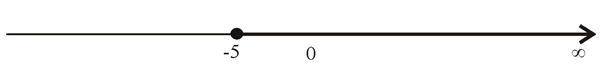# To express: the given inequality in terms of an interval and then graph the interval.### Precalculus: Mathematics for Calcu...

6th Edition
Stewart + 5 others
Publisher: Cengage Learning
ISBN: 9780840068071### Precalculus: Mathematics for Calcu...

6th Edition
Stewart + 5 others
Publisher: Cengage Learning
ISBN: 9780840068071

#### Solutions

Chapter 1.1, Problem 54E
To determine

## To express: the given inequality in terms of an interval and then graph the interval.

Expert Solution

In terms of interval the inequality can be expressed as [5,) .

### Explanation of Solution

Given information:

An inequalityis given as

x5

Concept used:

Aninequality a<x<b in terms of an interval can be expressed as (a,b) .

Aninequality axb in terms of an interval can be expressed as [a,b] .

Aninequality ax<b in terms of an interval can be expressed as [a,b) .

Aninequality a<xb in terms of an interval can be expressed as (a,b] .

Aninequality xb in terms of an interval can be expressed as (,b] .

Aninequality x<b in terms of an interval can be expressed as (,b) .

Aninequality xa in terms of an interval can be expressed as [a,) .

Aninequality x>a in terms of an interval can be expressed as (a,) .

Calculation:

Consider the given inequality.

x5

So, the inequality can be expressed as aninterval as [5,) .

Graph:

Now, graph the interval on number line as shown:Here, 5 is included to the interval, closed ball is placed for 5 on the number line.

Number line ends at .

### Have a homework question?

Subscribe to bartleby learn! Ask subject matter experts 30 homework questions each month. Plus, you’ll have access to millions of step-by-step textbook answers!